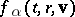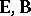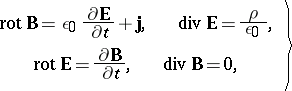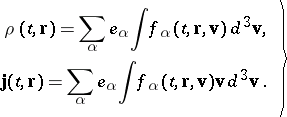# Vlasov kinetic equation

(diff) ← Older revision | Latest revision (diff) | Newer revision → (diff)

A kinetic equation for electrically-charged particles in which the interaction between the particles is described through a self-consistent electro-magnetic field. The equation has the form ,(1)

whereis the particle distribution function, while the indexis indicative for the kind of particle. The self-consistent electro-magnetic fieldfollows from the Maxwell equations(2)

in which the volume density of electric chargeand the volume density of electric currentare related to the particle distribution function via(3)

Vlasov's kinetic equation may be obtained from the Liouville equation for a distribution function of all particles of a given kindif either the particle interactions are neglected or it is assumed that the multi-particle distribution function is the product of single-particle distribution functions , .

The system of equations (1), (2), (3), proposed by A.A. Vlasov, is extensively employed in plasma physics. The linear theory, based on linearization of equations (1), (2), (3), is the most fully developed. It is used in the study of small oscillations and the stability of a plasma . The quasi-linear theory, which makes it possible to study non-linear effects, is in full development.

How to Cite This Entry:
Vlasov kinetic equation. Encyclopedia of Mathematics. URL: http://encyclopediaofmath.org/index.php?title=Vlasov_kinetic_equation&oldid=18325
This article was adapted from an original article by D.P. Kostomarov (originator), which appeared in Encyclopedia of Mathematics - ISBN 1402006098. See original article